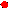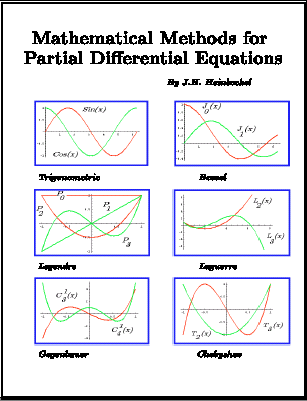OLD DOMINION UNIVERSITYCOLLEGE OF SCIENCESDEPARTMENT OF MATHEMATICS AND STATISTICS

A Partial Differential Equations Textbook

MATHEMATICAL METHODS FOR PARTIAL DIFFERENTIAL EQUATIONS

### FREE SAMPLE PAGES FROM EACH CHAPTERAn introductory partial differential equations textbook

and technical reference for Mathematicians, Engineers, Physicists and Scientists.

Topics Covered
Partial differential equations
Orthogonal functions
Fourier Series
Fourier Integrals
Separation of Variables
Boundary Value Problems
Laplace Transform
Fourier Transforms
Finite Transforms
Green's Functions
Special Functions
Sample pages from the textbook are available below.
There are numerous illustrative worked examples and answers to selected exercises are given.

If you like what you see you can order textbook through the following bookstores:
Trafford's Internet Bookstore.
Barnes and Noble
Amazon Books
Borders

If you have problems finding it, then give your local bookstore the following

International Standard Book Number.

ISBN 1-4120-0380-6

### SAMPLE PAGES

(Free samples of first 10 pages from start of each chapter)
CONTENTS.PDF
CHAPTER1.PDF
CHAPTER2.PDF
CHAPTER3.PDF
CHAPTER4.PDF
CHAPTER5.PDF
CHAPTER6.PDF
CHAPTER7.PDF
CHAPTER8.PDF
CHAPTER9.PDF
PDE TEXTBOOK is 576 pages

OLD DOMINION UNIVERSITYCOLLEGE OF SCIENCESDEPARTMENT OF MATHEMATICS AND STATISTICS